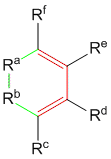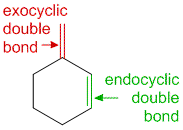# Ultraviolet-Visible (UV-Vis) Spectroscopy – Woodward-Fieser Rules to Calculate Wavelength of Maximum Absorption (Lambda-max) of Conjugated Dienes and Polyenes

## Introduction

As we have seen earlier in the chapter of absorption of a particular wavelength of light depends upon the π-electron system of the molecule. The more the conjugation of the π-electron system within the molecule, the higher the wavelength of light it can absorb. Robert Burns Woodward and Louis Fieser put down a set of rules which allows one to calculate the wavelength of maximum absorption (λmax) for a molecule empirically. These sets of rules to calculate the wavelength of maximum absorption or λmax of a compound in the ultraviolet-visible spectrum, based empirically have been called the Woodward-Fieser rules or Woodward’s-rules.

These sets of article aims to guide the student on how to use these rules to calculate the wavelength of maximum absorption or λmax for different systems. The following pages are listed for the readers.

Note: Numerical values for Woodward-Fieser rules differ slightly from one textbook to another. We have tried to compile an extensive list of numerical values from online resources, textbooks and journal articles based on the popularity of the number. It is recommended that you learn on how to apply the values for the contributors and then follow the values given in a text book recommended by your teacher, or use our values. We believe that learning how to apply the rules is more essential than actually getting the exact answer. Other’s opinions may vary.

## Woodward-Fieser Rules for Calculating the λmax of Conjugated Dienes and Polyenes

Conjugated dienes and polyenes are found in most organic compounds. For example, even a benzene ring is a conjugated polyene. Therefore it is useful to know how to utilize the Woodward-Fieser rules to calculate the wavelength of maximum absorption of conjugated dienes and polyenes.

According to Woodward’s rules the λmax of the molecule can be calculated using a formula:
λmax = Base value + Σ Substituent Contributions + Σ Other Contributions

Here the base value depends upon whether the diene is a linear or heteroannular or transoid diene, or whether it is a cyclic or homoannular diene (each of these will be explained in greater detail below). The sum of all substituent contributions are added to the base value to obtain the wavelength of maximum absorption of the molecule.

Table 1: Gives the values for the influence of different chromophores in conjugated diene systems as per Woodward-Fieser rules. The usage of these will become more evident in the examples which follow.

 Class Chromophore Influence to λmax Core Chromophore + 215 nmCisoid/Homoannular Diene + 253 nm (Note– Some text books mention it to be + 260 nm) Substituent Effects -R (Alkyl group) + 5 nm -OR (Alkoxy group) + 6 nm -X (-Cl, -Br, -halogen) + 10 nm -OC(O)R (Acyloxy/Ester) +  0 nm -SR (Sulfide) + 30 nm -NR2 (Amine) + 60 nm – C=C (double bond extending conjugation) + 30 nm – C6H5 (Phenyl group) + 60 nm Other ContributorsIf Exocyclic Double Bond (and not Endocyclic Double Bond) + 5 nm Solvent Effects Negligible

Let us discuss each of the above values and when to apply them in greater detail with examples:

### Core Chromophores With Base Values

1] Transoid Diene / Heteroannular diene / linear diene: This type of diene generally involves the attachement of two trans dienes together. Since the two double bonds attached are trans, it leads to a linear diene which is also called a heteroannular diene as the diene cannot be placed within one ring system as it would cause the ring to become very unstable (5 – 6 carbon rings are most stable with very few exceptions). The base value for a heteroannular diene system is 215 nm according to the Woodward-Fieser rules. Examples of heteroannular dienes are shown below.

In the above example, it can be seen that one of the double bonds belongs to ring A while the other double bond belongs to ring B, hence making the double bond heteroannular. Since both double bonds are trans with respect to substituents making the diene a transoid diene. In general, heteroannular dienes are transoid. If the diene is not a part of a ring then it is just transoid.

2] Cisoid diene / Homoannular diene / cyclic diene: This type of diene involves the conjugation of two cis dienes. Since the double bonds are cis to each other, the molecule often tends to form a closed ring system and therefore also called a cyclic or homoannular diene. The base value for homoannular diene system is 253 nm according to the Woodward-Fieser rules. Examples of homoannular dienes are shown below.

In the above example, it can be seen that both the double bonds belong to ring B making this type of diene a homoannular diene. Since both double bonds are cis with respect to substituents making the diene a cisoid diene. In general, homoannular dienes are cisoid. If the diene is not a part of a ring (i.e. the green bonds do not exist) then it is just a cisoid diene.

Important Note- If a molecule has both a homoannular diene and a heteroannular diene as the example 5 in the sample problems, then you must use the homoannular diene as the core chromophore.

### Substituent Effects

Only the substituents attached directly to the double bond diene systems can influence the ultraviolet visible absorption of the molecules. If the substituents are not directly attached to the carbons of the diene system, it will not affect the UV-Visible absorption spectrum of the molecule (as shown below).

The figure above highlights possible substituents in red given by the different -R groups. In the above examples 1 and 2, assignment of substituents must be given to all the atoms which are directly connected to the diene. Hence even though the structure has no substituents, the core carbon atoms have yet to be considered as alkyl-substituents. Hence in example 1, there are 3 alkyl substituents while in example 2, there are 4 alkyl substituents.

If one sees down the list of substituents an interesting factor in affecting the absorbance is whether the substituent directly has a heteroatom (with lone pairs) linked to the conjugated diene system. Below is the list of possible substituent effects.

 Substituent Influence -R (Alkyl group) + 5 nm -OR (Alkoxy group) + 6 nm -X (-Cl, -Br, -halogen) + 10 nm -OC(O)R (Acyloxy/Ester) +  0 nm -SR (Sulfide) + 30 nm -NR2 (Amine) + 60 nm – C=C (double bond extending conjugation) + 30 nm – C6H5 (Phenyl group) + 60 nm

Important Note- In case of double bond extending conjugation such as those shown in example 4 and example 5 in the sample problems page, the substituents on the extended double bond must also be counted for the calculation.

### Other Contributors

1] Exocylcic Double Bonds

Exocyclic doube bond by definition is a double bond where one of the participating carbon atoms is a part of a ring, while the other carbon atom is not part of the same ring. From the name we can understand that exo-cyclic would stand for a double bond outside the ring and endo-cyclic would stand for a double bond within the ring.

For each exocyclic double bond, we must add +5 nm to obtain the λmax. Below are a few examples as to what are exocyclic and what are endocyclic double bonds.

The above figure differentiates between exocyclic (shown in red) and endocyclic (shown in green) double bonds.

1. In example 1, the double bond present within ring A is exocyclic to ring B as it is attached to an atom which is shared between ring A and ring B, while the double bond present in ring B is not connected to any ring A atoms and is within just one ring, hence making it endocyclic.
2. In example 2, both double bonds are present within ring B with connections to shared carbon atoms with ring A, making both the double bonds exocyclic.
3. In example 3, there is a single double bond which is exocyclic at two points to two different rings. In such a case, the influence would be 2 times + 5 nm (i.e + 10 nm).

Note: Double bonds which are common to two rings are endocyclic. Below we give an example of a double bond which although has carbon atoms shared between two rings (A and B), it is considered endocyclic as at any given time the double bond will only belong to one ring.

2] Solvent effects

Since the conjugated diene base is relatively non-polar, contribution due to different solvents is very minor and can be ignored in most cases.

## Fieser-Kuhn Rules

Woodward-Fieser rules for dienes work well for conjugated systems with less than 4 double bonds in . For molecules having more than 4-double bonds, one must use the Fieser-Kuhn rules to determine the λmax. Check out this post for Fieser-Kuhn Rules to Calculate Wavelength of Maximum Absorption (Lambda-max) of Polyenes (with Sample Problems) to learn how to use these rules.

### Problems to Use Woodward-Fieser Rules for Calculating the λmax of Dienes.

Check out the page for  Also check out the page on

## Books on Analytical Chemistry and Spectroscopy

Check out these good books for analytical chemistry and spectroscopy

## References

1. Woodward, R. B. Structure and Absoprtion Spectra. III. Normal Conjugated Dienes. J. Am. Chem. Soc. 1942. 64(1), 72-75.
2. Woodward, R. B. Clifford, A. F. Structure and Absoprtion Spectra. II. 3-Acetoxy-Δ5-(6)-nor-cholestene-7-carboxylic Acid. J. Am. Chem. Soc. 1941. 63(10), 2727-2729.
3. Fieser, L. F., Fieser, M., Rajagopalan, S. Absorption spectroscopy and the structures of the diosterols. J. Org. Chem. 1948. 13(6), 800-806.
4. Spectroscopic Determination of Organic Compounds, 5th Edition, Silverstein, Bassler, Morrill 1991.
5. Kalsi, P. S. Spectroscopy of Organic Compounds. 6th Edition, New Age International Publishers, New Delhi, 2004.
6. Glagovich, N. Woodward Fieser Rules for Dienes. [Online]http://www.chemistry.ccsu.edu/glagovich/teaching/316/uvvis/diene.html (Accessed on July 27, 2012).
7. Author Unknown. Michigan State University – Department of Chemistry. Emperical Rules for absorption wavelengths of conjugated systems. [Online]http://www2.chemistry.msu.edu/faculty/reusch/VirtTxtJml/Spectrpy/UV-Vis/uvspec.htm (Accessed on July 27, 2012).

#### Related Posts

1.2.3.4.5.6.7.8.9.10.11.12.13.•14.15.16.17.18.19.•20.21.•22.•23.24.25.26.•27.•••28.29.•30.•31.32.••••